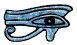# Triangles

A triangle has three straight line sides. The word triangle comes from a Latin word which means three-cornered. The corners of a triangle are called its vertices (singular vertex).

If all the sides of a triangle are different lengths it is a scalene triangle. We can show that if all the sides of a triangle are different then all the angles are different too, and visa versa.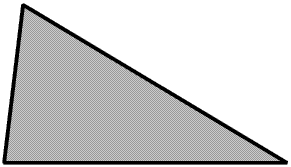If two of the sides are equal then it is an isosceles triangle, from the Greek for equal legs.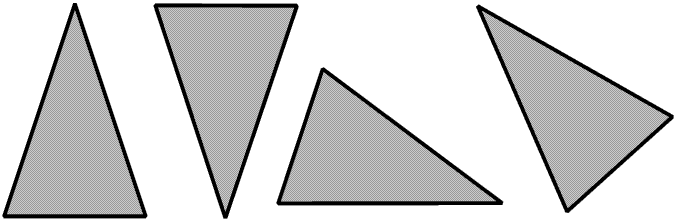We often draw an isosceles triangle with the unequal side at the bottom, as at the left of the picture, but it does not have to be like that, it can be at any angle.

We can show that if two of the sides of a triangle are equal then so are two of the angles, and visa versa.

If all three sides of a triangle are equal then it is an equilateral triangle, from the Latin for equal length.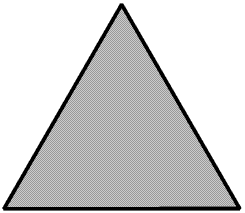We can show that if all the sides of a triangle are equal then so are all the angles, and visa versa. We show later on this Page that each of the angles in an equilateral triangle is 60°.

The angles inside a triangle are called the internal angles. If we extend one side we form the external angle. The internal and external angles at any vertex of a triangle (or any polygon) are supplementary, that is, they add up to 180° (two right angles). Two angles are complementary if they add up to 90° (one right angle.) When we are talking about the angles of a triangle we usually mean the internal angles, we do not usually need to say internal angle unless there might be some confusion as to whether we mean internal or external angle.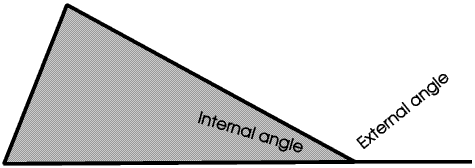If we walk along one side of a large triangle drawn on the ground, when we come to a vertex the external angle is the angle we must turn through in order to be able to walk along the next side.

For an equilateral triangle the internal angles are all equal, and as the internal and external angles are supplementary, the external angles must also all be equal.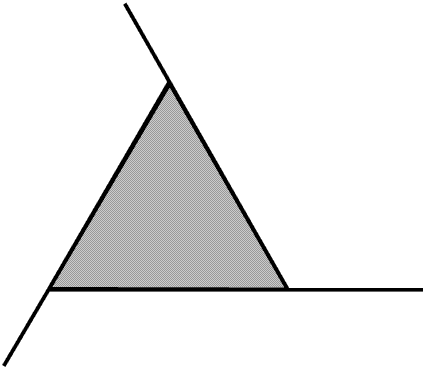If we walk all round an equilateral triangle so that we end up where we started from and are facing in the same direction we shall have turned through a complete circle of 360°. This means that the external angles add up to 360° so each one of them must be 360° ÷ 3 or 120°. As the external and internal angles are supplementary each internal angle must be 180° - 120° or 60°, so the sum of the internal angles is 3 × 60° or 180°. We can show that this is true for all triangles, not only equilateral triangles, that is,

For any triangle the sum of the internal angles is 180°.

This means that if one of the (internal) angles in a triangle is 90° or more then the sum of the other two angles must be 90° or less, that is, a triangle can have only one right angle or one obtuse angle. In an acute angled triangle all the angles are acute, in a right angled triangle one angle is a right angle, in an obtuse angled triangle one angle is obtuse.

We can combine these terms with scalene and isosceles, so the different types of triangle are
• Acute angled scalene
• Right angled scalene
• Obtuse angled scalene
• Acute angled isosceles
• Right angled isosceles
• Obtuse angled isosceles
• Equilateral - this must be acute angled so we do not need to say so.
There is more about right angled triangles on the Right Angled Triangle Page of my Web Site (currently under construction).

We usually label the vertices of a triangle or other polygon and the ends of a straight line with capital letters, often consecutive letters, such as A, B and C or P, Q and R.Here the sides are PQ, QR and PR. (The order of the letters, for example PR or RP, matters only if the sides of the triangle are vectors - this is not discussed further here.)

The whole triangle is called triangle PQR; the angle between PQ and PR can be called angle QPR, or if there cannot be any confusion what angle is meant just angle P. The angle P is included between PQ and PR, and (angle) P and (side )QR are opposite.

We often use small letters to stand for the lengths of the sides. Conventionally, the length of the side opposite angle (big) P is given the symbol (little) p. Remember that p stands for the length of the side not the side itself: we must not refer to side p, only side QR. The longest side is always opposite the largest angle.

If we have three rods we can join them at their ends to make a triangle. The only Rule is that any two of the rods must together be longer than the third, otherwise their ends will not join.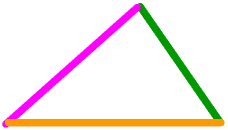There is only one way we can join these rods: the brown one will always have a magenta rod at one end and a green rod at the other.

If you are colour-blind, and in England about one boy in ten is, I have tried to choose colours where you can see the difference, but if you cannot please e-mail me and I will change them.

Of course, you can turn the shape round or upside down in any way you like but it will always be exactly the same size and shape.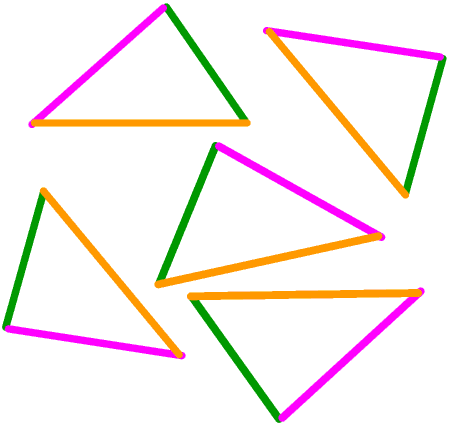This is very important because it means that once we have fixed the length of the sides of a triangle we have also fixed the angles. This is always true for all triangles, it is never true for any other polygon.

Triangles which are exactly the same size and shape are congruent.

Going on from here, if we have a triangle and increase the length of just one side by a certain proportion, say 80%, while keeping the angles the same we also increase the length of the other two sides by 80%.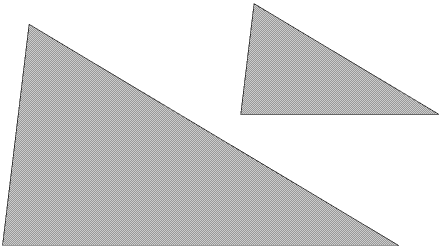The two triangles are the same shape but not the same size. This is always true of all triangles; it is never true for any other polygon. Triangles which are the same shape but not the same size are similar.

These important differences between triangles and all other polygons are discussed at greater length in The Shape of a Polygon.

For all triangles, the ratio of the length of the sides depends only upon the size of the angles, and the size of the angles depends only on the ratio of the length of the sides. A whole branch of mathematics, called trigonometry, is founded on this very simple idea.

## The area of a triangleHere triangle ABF is half the area of the rectangle ADBF, and triangle FBC is half the area of rectangle FBEC. So triangle ABC is half the area of the rectangle ADEC. The area of rectangle ADEC is base × height so the area of triangle ABC is ½ (base × height).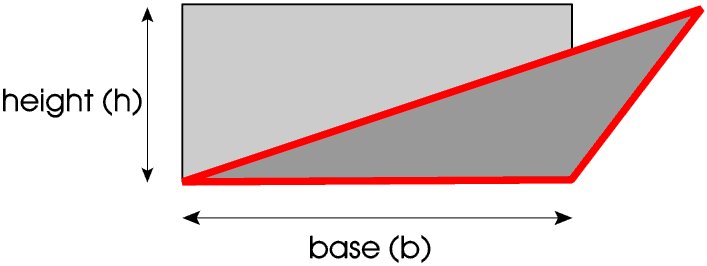The apex of the triangle does not have to lie on the rectangle for this to be true. You might like to try to prove this for yourself - it is not difficult. But e-mail me if you get stuck.

The area of any triangle is half the base times the (vertical) height.

© Barry Gray May 2007